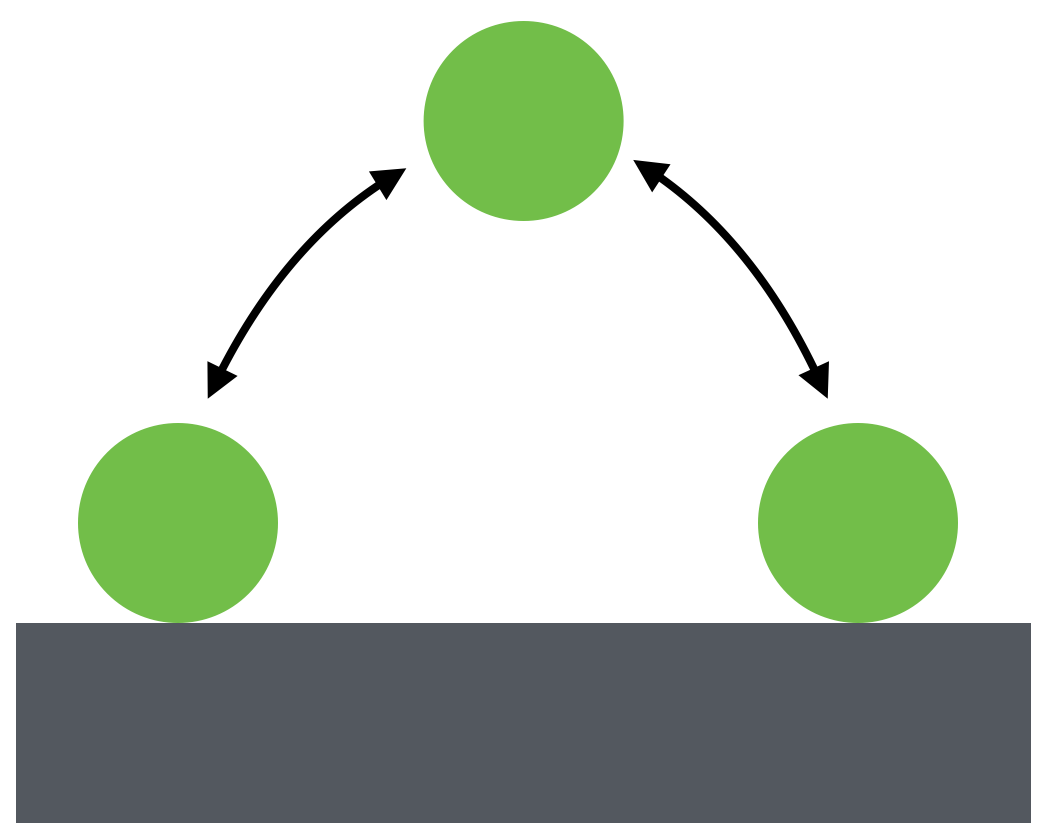# There and back againSuppose you want to throw a super ball so that it bounces back and forth, retracing the same trajectory, as shown above. If you throw the ball horizontally with velocity $\SI[per-mode=symbol]{1}{\meter\per\second},$ what spin $\omega$ (in $\si[per-mode=symbol]{\radian\per\second}$) must you give it so that it does what you want?

Assumptions and Details

• The collision with the ground is perfectly elastic.
• The ball does not slip while it contacts the ground.
• The radius of the ball is $\SI{3}{\centi\meter}.$
×